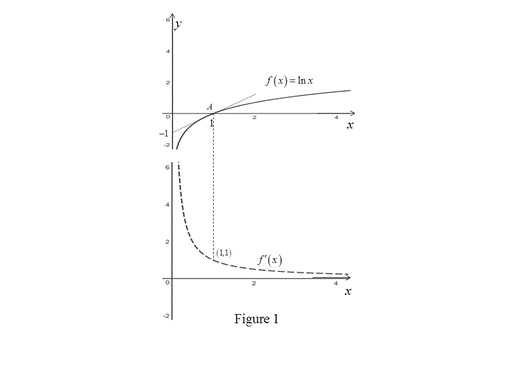# The graph of f ( x ) and then f ′ ( x ) below it.### Single Variable Calculus: Concepts...

4th Edition
James Stewart
Publisher: Cengage Learning
ISBN: 9781337687805### Single Variable Calculus: Concepts...

4th Edition
James Stewart
Publisher: Cengage Learning
ISBN: 9781337687805

#### Solutions

Chapter 2.7, Problem 16E
To determine

## To Sketch: The graph of f(x) and then f′(x) below it.

Expert Solution

The formula for f(x) from its graph is, f(x)=1x for all x>0.

### Explanation of Solution

The given function is f(x)=lnx.

From the graph of f(x)=lnx, it is clear that the slope is always positive.

Thus, the derivative of the graph is always positive.

Draw the tangent to the graph at x=1 and the slope of the graph at x=1 is computed as follows,

m=0110=11=1

Recall the fact that, the slope of the function at the point x=a is equal to the derivative of the function at that point.

Therefore, f(x)=1 at x=1.

Thus, the graph of the function f(x) passes through the point (1, 1).

From the point A to left, the slope decreases rapidly. Thus, the graph of the function f(x) in this section will decrease rapidly.

From the point A to right, the slope is increasing slowly. Thus, the graph of the function f(x) in this section will decrease slowly.

Graph:

Use the online graphing calculator to draw the graph of f(x)=lnx and use the above information to trace the graph of f(x) as shown below in Figure 1.Observation:

From Figure 1, it is observed that the graph of the derivative function seems to be 1x for x>0.

Thus, the derivative formula is, f(x)=1x for all x>0.

### Have a homework question?

Subscribe to bartleby learn! Ask subject matter experts 30 homework questions each month. Plus, you’ll have access to millions of step-by-step textbook answers!